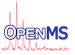OpenMS  2.8.0
IsoSpecTotalProbWrapper Class Reference

Create a p-set of configurations for a given p (that is, a set of configurations such that their probabilities sum up to p). The p in normal usage will usually be close to 1 (e.g. 0.99). More...

`#include <OpenMS/CHEMISTRY/ISOTOPEDISTRIBUTION/IsoSpecWrapper.h>`

Inheritance diagram for IsoSpecTotalProbWrapper:
[legend]
Collaboration diagram for IsoSpecTotalProbWrapper:
[legend]

## Public Member Functions

IsoSpecTotalProbWrapper (const std::vector< int > &isotopeNumbers, const std::vector< int > &atomCounts, const std::vector< std::vector< double > > &isotopeMasses, const std::vector< std::vector< double > > &isotopeProbabilities, double p, bool do_p_trim=false)
Constructor. More...

IsoSpecTotalProbWrapper (const IsoSpecTotalProbWrapper &)=delete

IsoSpecTotalProbWrapper (const EmpiricalFormula &formula, double p, bool do_p_trim=false)
Setup the algorithm to run on an EmpiricalFormula. More...

~IsoSpecTotalProbWrapper ()

IsotopeDistribution run () final
Run the algorithm. More...Public Member Functions inherited from IsoSpecWrapper
virtual ~IsoSpecWrapper ()=default

## Protected Attributes

std::unique_ptr< IsoSpec::IsoLayeredGenerator > ILG

const double target_prob

const bool do_p_trim

## Detailed Description

Create a p-set of configurations for a given p (that is, a set of configurations such that their probabilities sum up to p). The p in normal usage will usually be close to 1 (e.g. 0.99).

An optimal p-set of isotopologues is the smallest set of isotopologues that, taken together, cover at least p of the probability space (that is, their probabilities sum up to at least p). This means that the computed spectrum is accurate to at least degree p, and that the L1 distance between the computed spectrum and the true spectrum is less than 1-p. The optimality of the p-set means that it contains the most probable configurations - any isotopologues outside of the returned p-set have lower intensity than the configurations in the p-set.

This is the method most users will want: the p parameter directly controls the accuracy of results.

Advanced usage note: The algorithm works by computing an optimal p'-set for a p' slightly larger than the requested p. By default these extra isotopologues are returned too (as they have to be computed anyway). It is possible to request that the extra configurations be discarded, using the do_p_trim parameter. This will *increase* the runtime and especially the memory usage of the algorithm, and should not be done unless there is a good reason to.

Note
The eligible configurations are NOT guaranteed to be returned in any particular order.

## ◆ IsoSpecTotalProbWrapper() [1/3]

 IsoSpecTotalProbWrapper ( const std::vector< int > & isotopeNumbers, const std::vector< int > & atomCounts, const std::vector< std::vector< double > > & isotopeMasses, const std::vector< std::vector< double > > & isotopeProbabilities, double p, bool do_p_trim = `false` )

Constructor.

Parameters
 isotopeNumbers A vector of how many isotopes each element has, e.g. [2, 2, 3]) atomCounts How many atoms of each we have [e.g. 12, 6, 6 for Glucose] isotopeMasses Array with the individual elements isotopic masses isotopeProbabilities Array with the individual elements isotopic probabilities p Total coverage of probability space desired, usually close to 1 (e.g. 0.99) do_p_trim Whether to discard extra configurations that have been computed
Note
This constructor is only useful if you need to define non-standard abundances of isotopes, for other uses the one accepting EmpiricalFormula is easier to use.

## ◆ IsoSpecTotalProbWrapper() [2/3]

 IsoSpecTotalProbWrapper ( const IsoSpecTotalProbWrapper & )
delete

## ◆ IsoSpecTotalProbWrapper() [3/3]

 IsoSpecTotalProbWrapper ( const EmpiricalFormula & formula, double p, bool do_p_trim = `false` )

Setup the algorithm to run on an EmpiricalFormula.

## ◆ ~IsoSpecTotalProbWrapper()

 ~IsoSpecTotalProbWrapper ( )

## ◆ run()

 IsotopeDistribution run ( )
finalvirtual

Run the algorithm.

This method will run the algorithm with parameters as set up by the constructor. It will return an IsotopeDistribution containing the observed configurations. The configurations are explicitly stored in memory, which may become a problem when considering some especially large distributions. If this, or (a rather small) performance overhead is a concern, then the generator methods (see IsoSpecGeneratorWrapper) should be used instead.

This method is provided for convenience. As calling that method invalidates the object (the method should not be called again, nor anything other than destroying the object should be done with it), the most common usage pattern of IsoSpecGeneratorWrapper classes with the run method is:

IsotopeDistribution dist = IsoSpecGeneratorWrapperSubclass(...).run();
// do something with dist;
Note
Calling this method invalidates the object! In future versions this limitation might be removed.

Implements IsoSpecWrapper.

## ◆ do_p_trim

 const bool do_p_trim
protected

## ◆ ILG

 std::unique_ptr ILG
protected

## ◆ target_prob

 const double target_prob
protected